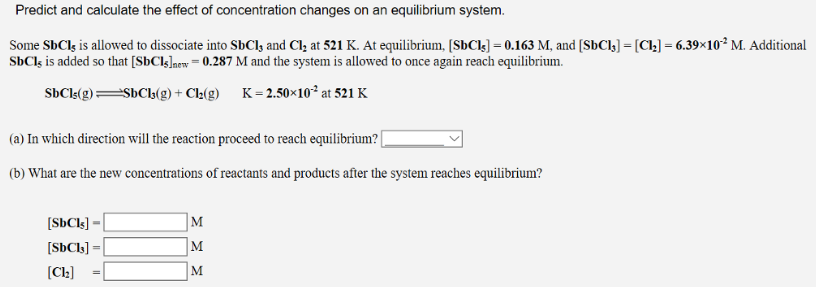# Problem: Predict and calculate the effect of concentration changes on an equilibrium system. Some SbCl5 is allowed to dissociate into SbCl3, and Cl2 at 521 K. At equilibrium, [SbCl5] = 0.163 M, and [SbCl3] = [Cl2] = 6.39 x 10-2 M. Additional SbCl5 is added so that [SbCl5]new = 0.287 M and the system is allowed to once again reach equilibrium. SbCl5 (g) ⇌ SbCl3 (g) + Cl2 (g) K = 2.50 x 10-2 at 521 K (a) In which direction will the reaction proceed to reach equilibrium? (b) What are the new concentrations of reactants and products after the system reaches equilibrium?

###### FREE Expert Solution
97% (415 ratings)###### Problem Details

Predict and calculate the effect of concentration changes on an equilibrium system.

Some SbCl5 is allowed to dissociate into SbCl3, and Cl2 at 521 K. At equilibrium, [SbCl5] = 0.163 M, and [SbCl3] = [Cl2] = 6.39 x 10-2 M. Additional SbCl5 is added so that [SbCl5]new = 0.287 M and the system is allowed to once again reach equilibrium.

SbCl(g) ⇌ SbCl3 (g) + Cl2 (g) K = 2.50 x 10-2 at 521 K

(a) In which direction will the reaction proceed to reach equilibrium?

(b) What are the new concentrations of reactants and products after the system reaches equilibrium?